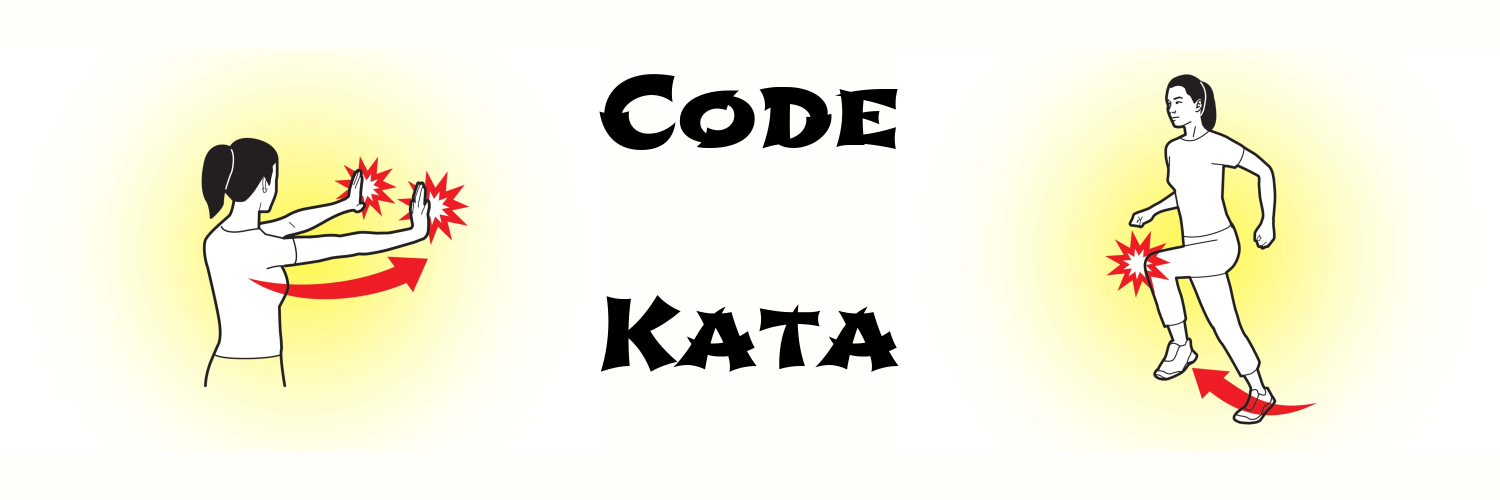# Programming Kata Day 1From today, I will practice one programming kata per day and post the content here.

Here goes the first one:

My friend John likes to go to the cinema. He can choose between system A and system B.

System A : buy a ticket (15 dollars) every time

System B : buy a card (500 dollars) and every time buy a ticket the price of which is 0.90 times the price he paid for the previous one.

Example:

System A : 15 * 3 = 45

System B : 500 + 15 * 0.90 + (15 * 0.90) * 0.90 + (15 * 0.90 * 0.90) * 0.90 ( = 536.5849999999999, no rounding for each ticket)

John wants to know how many times he must go to the cinema so that the final result of System B, when rounded up to the next dollar, will be cheaper than System A.

(defn movie [card ticket perc]
(loop [n 1
b-price (* ticket perc)]
(if (< (Math/ceil (+ card b-price)) (* ticket n))
n
(recur (inc n) (+ b-price (* ticket (Math/pow perc (inc n))))))))